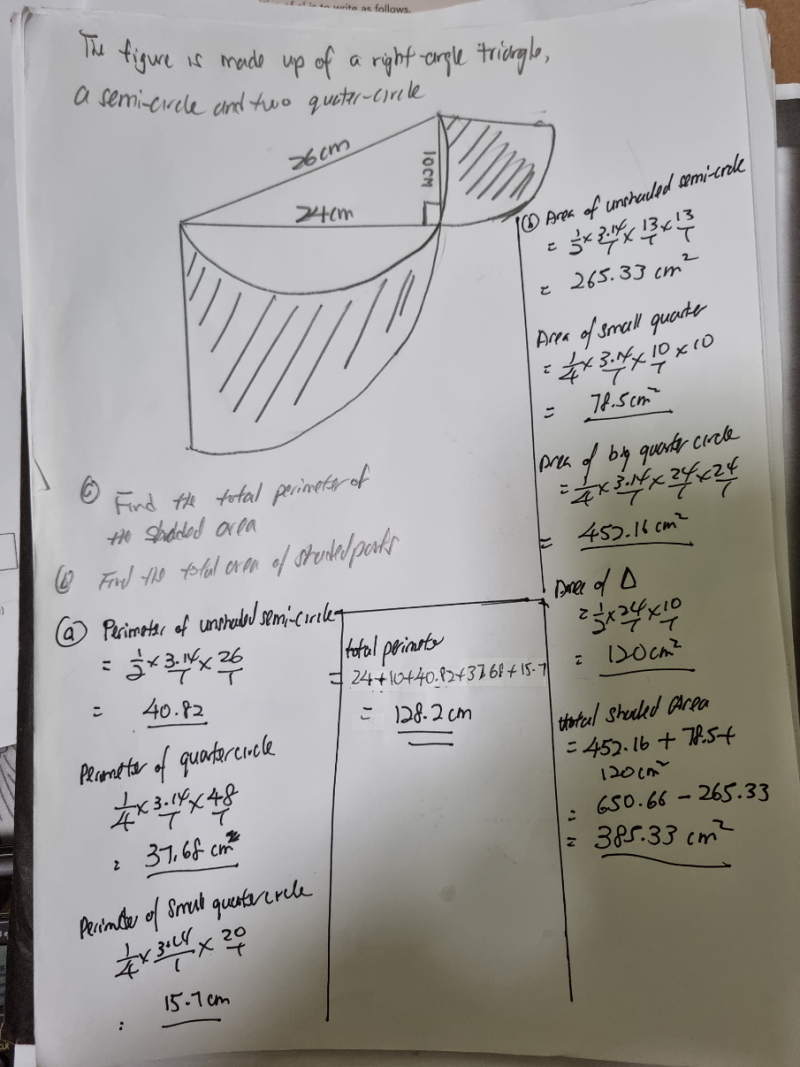# QuestionIt should be:

Area of total figure = 452.16 + 78.5 + 120 = 650.66

Hence Area of shaded part = Area of total figure – Area of semi circle = 650.66 -265.33 = 385.33cm2

Your presentation of the final part is incorrect:

Area of shaded part = 452.16 + 78.5 + 120 = 650.66 -265.33 = 385.33cm2  is technically wrong because 452.16 + 78.5 + 120  is not equal to 650.66 -265.33

Do not join the calculation this way, either split into above like what I have written or put everything in one line

452.16 + 78.5 + 120 – 265.33 = 385.33cm2

0 Replies 2 Likes ✔Accepted Answer